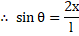# A small ball of massis attached with upper end of a uniform straight rod of equal massand lengthThe rod is held vertical over a smooth horizontal surface as shown in fig. When the system is released, the lower end slips freely and the systems falls down. Assuming the initial position of the lower end to be origin and initially rod to be along-axis as shown in fig., the equation of trajectory of pointof the rod (is at distancefrom the lower end)isa)b)c)d)## Question ID - 100141 :- A small ball of massis attached with upper end of a uniform straight rod of equal massand lengthThe rod is held vertical over a smooth horizontal surface as shown in fig. When the system is released, the lower end slips freely and the systems falls down. Assuming the initial position of the lower end to be origin and initially rod to be along-axis as shown in fig., the equation of trajectory of pointof the rod (is at distancefrom the lower end)isa)b)c)d)3537

(b)

Since the floor is smooth, therefore no horizontal force is acting on the system. Only three forces are acting on it, weightof the ball, weightof the rod and vertically upward reaction of the floorSince the rod is released from rest and no horizontal force is acting on it, therefore centre of mass of the system will not displace horizontally; it means it will fall vertically downward

Since masses of the rod and ball are equal, therefore centre of mass ‘’ is at mid-point of centre of the rod and the ball; it means at distancefrom the ball or 3/4 from the lower end

If at an instant the rod makes anglewith the vertical, then pointand the system will be as shown in the figure

Co-ordinates of the pointareUsingwe haveNext Question :
 In concave mirror the object is placed beyond C. Then the nature and size of the image formed is (a) Real, erect and diminished (b) Real, inverted and diminished (c) Virtual, erect and magnified (d) Real, inverted and magnified